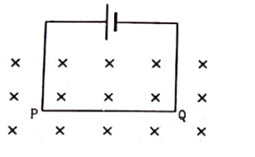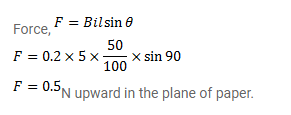# A current of 5.0A exists in the circuit shown in figure.

Question:

A current of $5.0 \mathrm{~A}$ exists in the circuit shown in figure. The wire $P Q$ has a length of $50 \mathrm{~cm}$ and the magnetic field in which it is immersed has a magnitude of $0.20 \mathrm{~T}$. Find the magnetic force acting on the wire $\mathrm{PQ}$.Solution: